Question
asap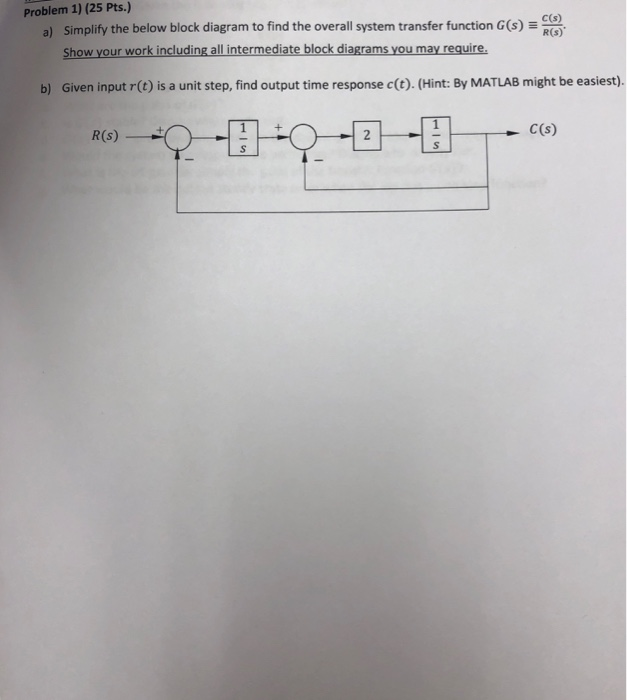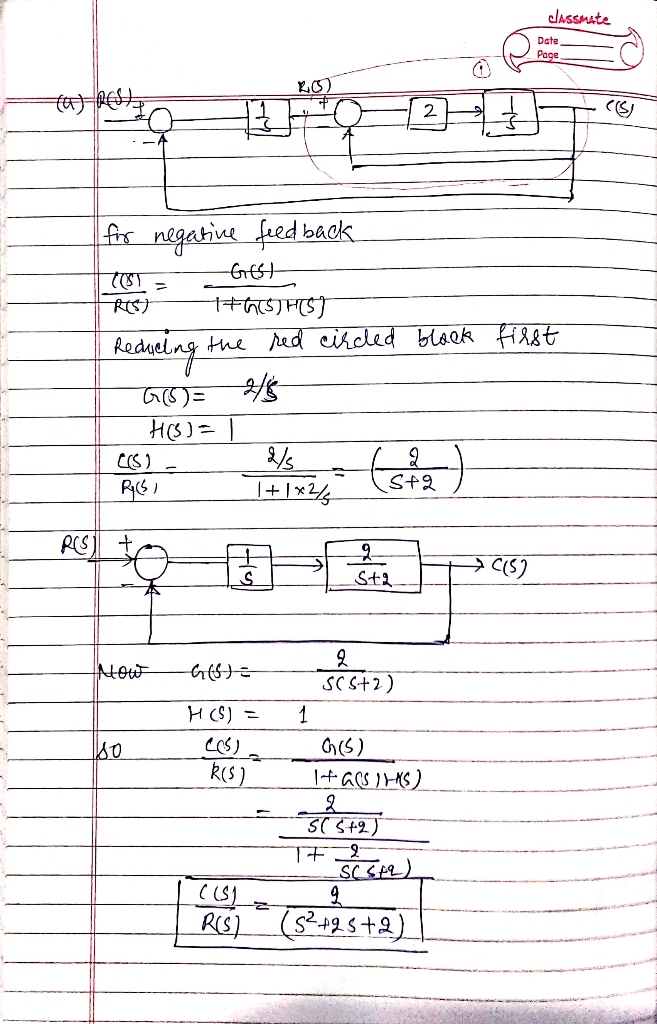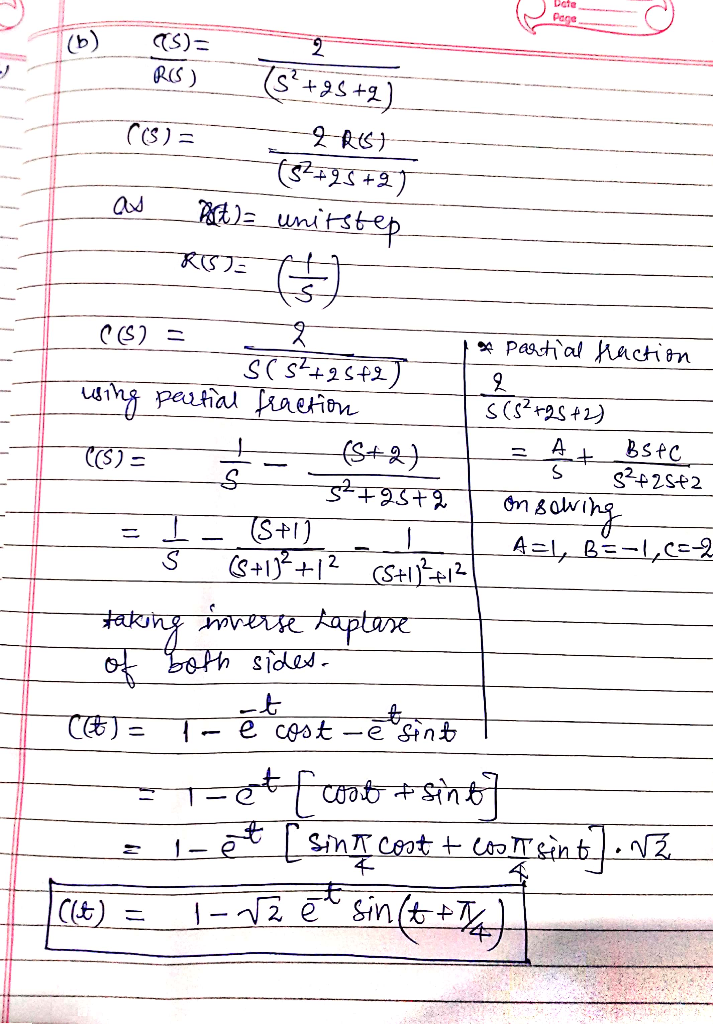#### Earn Coins

Coins can be redeemed for fabulous gifts.

Similar Homework Help Questions
• ### Question #2 ( 25 points) C(s) a) Reduce the block diagram shown in Figure 1 to a single transfer function T(s) =R)...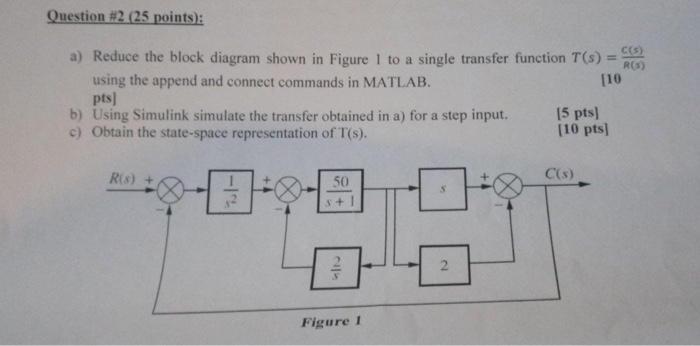Question #2 ( 25 points) C(s) a) Reduce the block diagram shown in Figure 1 to a single transfer function T(s) =R) using the append and connect commands in MATLAB. pts b) Using Simulink simulate the transfer obtained in a) for a step input. c) Obtain the state-space representation of T(s). [10 [5 pts [10 pts] C(s) Ris 50 s+I 2 Figure 1 -Irt Question #2 ( 25 points) C(s) a) Reduce the block diagram shown in Figure 1 to...

• ### Problem 4) (20 Pts.) A Proportional controller is simply a gain block. In figure below, it...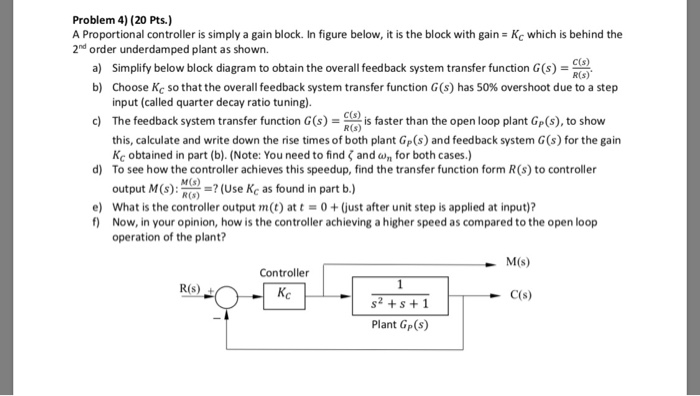Problem 4) (20 Pts.) A Proportional controller is simply a gain block. In figure below, it is the block with gain 2nd order underdamped plant as shown. Kc which is behind the a) Simplify below block diagram to obtain the overall feedback system transfer funion)R(G) b) Choose Kc so that the overall feedback system transfer function G(s) has 50% overshoot due to a step input (called quarter decay ratio tuning) d) The feedback system transfer function Gs)- is faster than...

• ### Problem 1: Consider the following block diagrams: a. Find the system transfer function C(s)/R(S). Simplify as...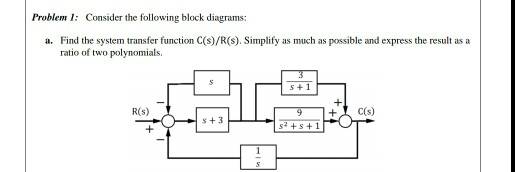Problem 1: Consider the following block diagrams: a. Find the system transfer function C(s)/R(S). Simplify as much as possible and express the result as a ratio of two polynomials.

• ### [HELP!] 1. Simplify the following block diagram. Obtain the transfer function from R to C for Fig. 1, and the transfer function from X(s) to Y(s) for Fig. 2. 2. Convert the block diagram of figures 1 and 2 to a signal flow graph.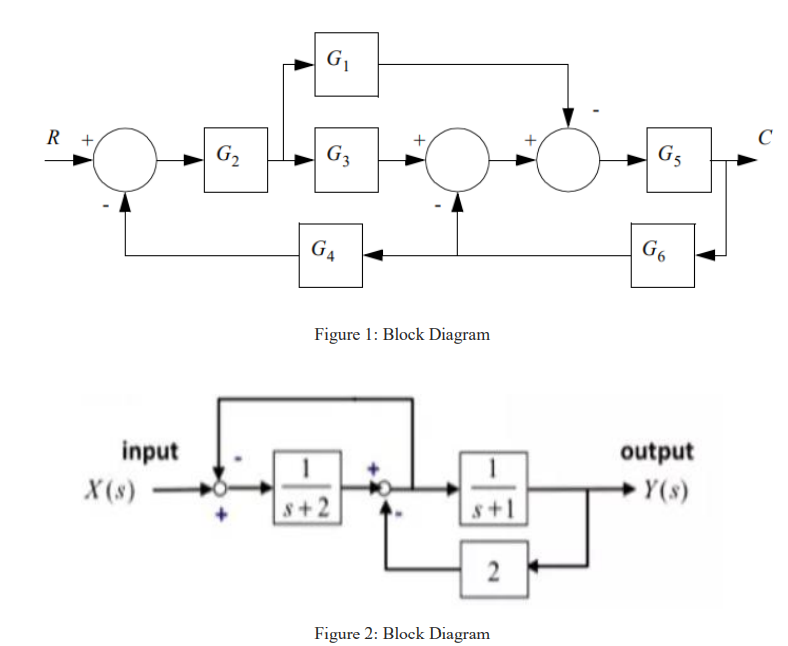Simplify the following block diagram. Obtain the transfer function from R to C for Fig. 1, and the transfer function from X(s) to Y(s) for Fig. 2.Convert the block diagram of figures 1 and 2 to a signal flow graph.Below are the diagrams:

• ### In the block diagram of the feedback control system shown in figure below, Gp(s) is the...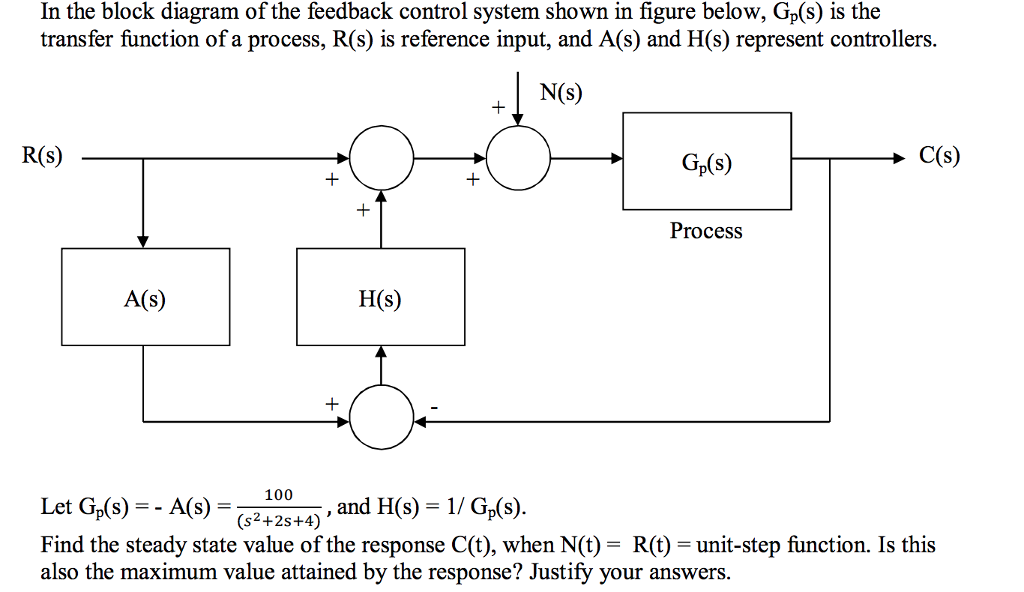In the block diagram of the feedback control system shown in figure below, Gp(s) is the transfer function of a process, R(s) is reference input, and A(s) and H(s) represent controllers. N(S) R(s) Gp(s) Process A(s) H(s) = _100_ , and H(s)-1 / GAS). Let Gs)-A(S)5.and Find the steady state value of the response C(t), when N(t) = R(t) = unit-step function. Is this also the maximum value attained by the response? Justify your answers. (s2+2s+4)

• ### 3. (10 points) Simplify the following block diagram and obtain the transfer function from s) to Y(s) input output +...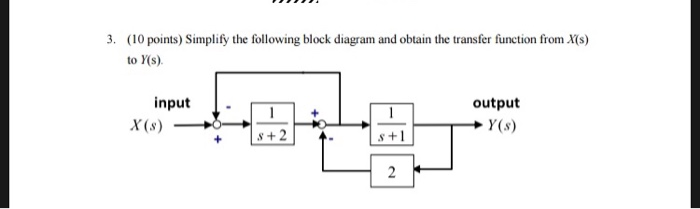3. (10 points) Simplify the following block diagram and obtain the transfer function from s) to Y(s) input output + Y(s) X(s) 8+2 s+ 2 3. (10 points) Simplify the following block diagram and obtain the transfer function from s) to Y(s) input output + Y(s) X(s) 8+2 s+ 2

• ### 1. 4 R(S) 1 s 1 S C(s) b) A system has a block diagram as...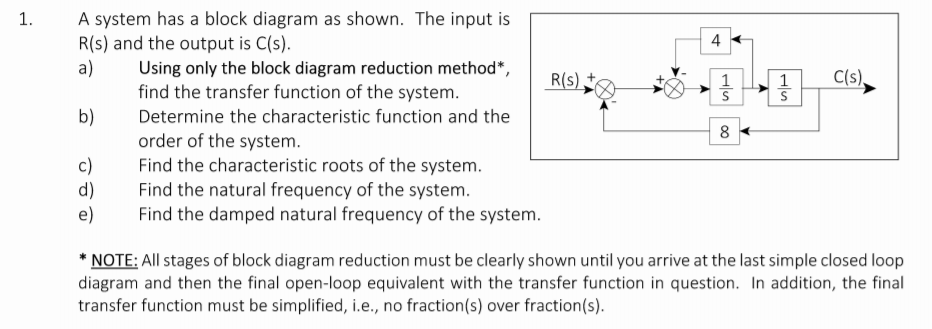1. 4 R(S) 1 s 1 S C(s) b) A system has a block diagram as shown. The input is R(s) and the output is C(s). a) Using only the block diagram reduction method*, find the transfer function of the system. Determine the characteristic function and the order of the system c) Find the characteristic roots of the system. Find the natural frequency of the system. e) Find the damped natural frequency of the system. 8 *NOTE: All stages of...

• ### 1. 4 C(s), S S A system has a block diagram as shown. The input is...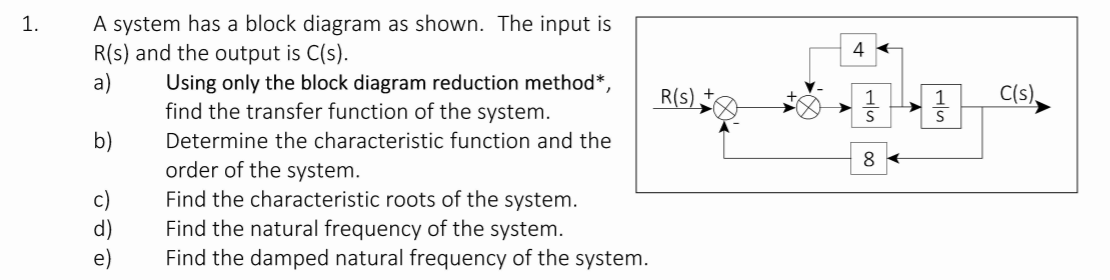1. 4 C(s), S S A system has a block diagram as shown. The input is R(s) and the output is C(s). a) Using only the block diagram reduction method*, R(s) find the transfer function of the system. b) Determine the characteristic function and the order of the system. Find the characteristic roots of the system. d) Find the natural frequency of the system. e) Find the damped natural frequency of the system. 8

• ### 2. The block diagram below model a simple DC motor for speed control application. Input V(s)...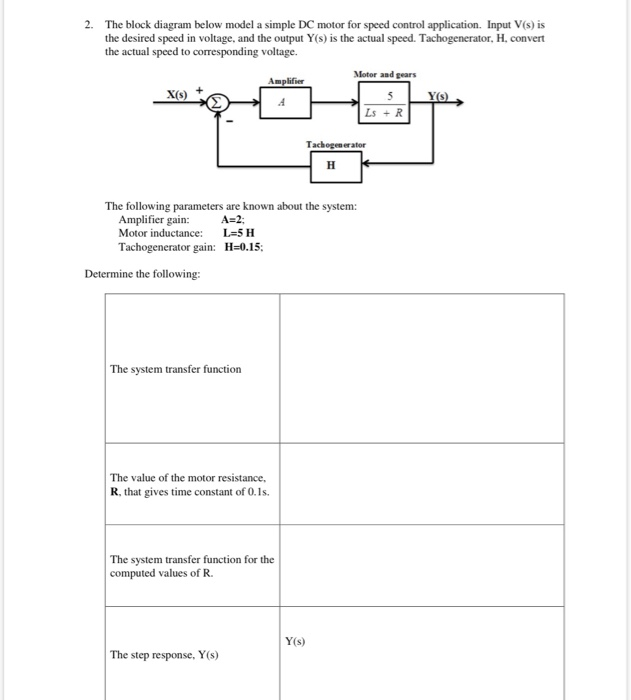2. The block diagram below model a simple DC motor for speed control application. Input V(s) is the desired speed in voltage, and the output Y(s) is the actual speed. Tachogenerator, H, convert the actual speed to corresponding voltage. Amplifier Motor and gears X() 5 Ls +R Tachogenerator H The following parameters are known about the system: Amplifier gain: A=2; Motor inductance: L=5H Tachogenerator gain: H=0.15; Determine the following: The system transfer function The value of the motor resistance, R,...

• ### 4 C(s) حرام | A system has a block diagram as shown. The input is "R(s)...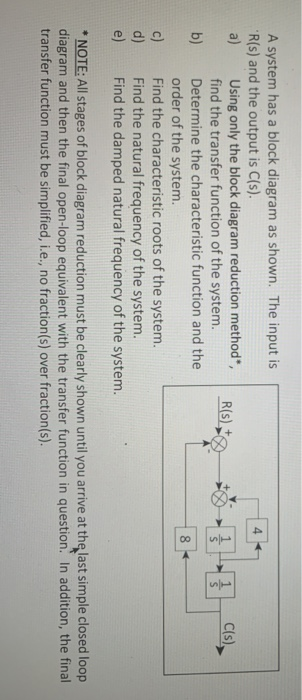4 C(s) حرام | A system has a block diagram as shown. The input is "R(s) and the output is C(s). a) Using only the block diagram reduction method, R(S) find the transfer function of the system. b) Determine the characteristic function and the order of the system c) Find the characteristic roots of the system. d) Find the natural frequency of the system. e) Find the damped natural frequency of the system. 8 * NOTE: All stages of block...